Published in Marine 2011, IV Int. Conference on Computational Methods in Marine Engineering. Selected papers, L. Eça, E. Oñate, J. García-Espinosa, T. Kvamsdal and P. Bergan (Eds.), pp. 65-81, Springer, 2013
DOI: 10.1007/978-94-007-6143-8_4

Abstract

We present some developments in the Particle Finite Element Method (PFEM) for the solution of complex coupled problems in marine, naval and harbour engineering involving fluid-soil-structure interaction (FSSI). The PFEM uses an updated Lagrangian description to model the motion of nodes (particles) in a continuum domain containing fluid, soil/rock and structures subdomains. A mesh connects the nodes defining the discretized domain where the governing equations for each of the constituent materials are solved with the FEM. The stabilization for dealing with an incompressibility material is introduced via the finite calculus (FIC) method. An incremental iterative scheme for solving the non linear transient FSSI problem is described. The procedure to model frictional contact conditions and material erosion at fluid-solid and solid-solid interfaces is described. We present examples of application of the PFEM to solve FSSI problems in marine, naval and harbour engineering such as the motion of rocks by water streams, the stability of breakwaters and constructions under sea waves, the sinking of ships and the collision of a ship with ice blocks.

1 Introduction

The analysis of problems involving the interaction of fluids, soil/rocks and structures is relevant in many areas of marine, naval and harbour engineering. Some representative examples are the study of off-shore and harbour structures under large waves, constructions hit by tsunamis, erosion of sea bed and landscape adjacent to sea shore, motion of ships under severe sea conditions, the simulation of the sinking of a ship, the collision of a ship with ice blocks, excavation and drilling problems in the sea in petroleum and gas engineering, etc.

The authors have developed in previous works a particular class of Lagrangian formulation for solving problems involving complex interactions between (free surface) fluids and solids. The so-called the particle finite element method ([PFEM]), treats the mesh nodes in the fluid and solid domains as particles which can freely move and even separate from the main fluid domain representing, for instance, the effect of water drops. A mesh connects the nodes discretizing the domain where the governing equations are solved using a stabilized FEM.

An advantage of the Lagrangian formulation is that the non-linear and non symmetric convective terms disappear from the fluid equations [1,2]. The difficulty is however transferred to the problem of adequately (and efficiently) moving the mesh nodes. The theory and applications of the PFEM are reported in [1-18].

The solution of a FSSI problem requires solving the momentum and mass balance equations for the underlying continuum that may include incompressible domains (as it is the case for a fluid and some soils). In our work we use a stabilized mixed FEM based on Finite Calculus (FIC) which allows us to solve incompressible continua with a linear approximation for the velocity and pressure variables [6,9,18,20-23].

The layout of the chapter is as follows. In the next section the key ideas of the PFEM are outlined. Next the basic equations for a general continuum using a Lagrangian description and the FIC formulation are schematically presented. Then an algorithm for the transient solution is briefly described. The treatment of the coupled FSSI problem and the method for mesh generation and for identification of the free surface nodes are outlined. The procedure for treating the frictional contact interaction between fluid, soil and structure interfaces is explained. We present several examples of application of the PFEM to solve FSSI problems in marine, naval and harbour engineering such as the motion of rocks by water streams, the stability of breakwaters and constructions hit by sea waves, the study of the sinking of ships and the collision of a ship with ice blocks.

2 The basis of the particle finite element method

Let us consider a domain containing both fluid and solid subdomains (the solid subdomain may include soil/rock materials and/or structural elements). The moving fluid particles interact with the solid boundaries, thereby inducing the deformation of the solid which in turn affects the flow motion and, therefore, the problem is fully coupled.

In the PFEM both the fluid and the solid domains are modelled using an updated Lagrangian formulation . That is, all variables are assumed to be known in the current configuration at time $t$ . The new set of variables in both domains is sought for in the next or updated configuration at time $t+\Delta t$ . The finite element method (FEM) is used to solve the equations of continuum mechanics for each of the subdomains. Hence a mesh discretizing these domains must be generated in order to solve the governing equations for each subdomain in the standard FEM fashion [24-27].

The quality of the numerical solution depends on the discretization chosen as in the standard FEM. Adaptive mesh refinement techniques can be used to improve the solution.

2.1 Basic steps of the PFEM

For clarity purposes we will define the collection or cloud of nodes (${\textstyle C}$) pertaining to the fluid and solid domains, the volume (${\textstyle V}$) defining the analysis domain for the fluid and the solid and the mesh (${\textstyle M}$) discretizing both domains.

A typical solution with the PFEM involves the following steps.

1. The starting point at each time step is the cloud of points in the fluid and solid domains. For instance ${}^{n}C$ denotes the cloud at time $t=t_{n}$ (Figure 1).
2. Identify the boundaries for both the fluid and solid domains defining the analysis domain ${}^{n}V$ in the fluid and the solid. This is an essential step as some boundaries (such as the free surface in fluids) may be severely distorted during the solution, including separation and re-entering of nodes. The Alpha Shape method  is used for the boundary definition.
3. Discretize the fluid and solid domains with a finite element mesh ${}^{n}M.$ We use an effect mesh generation scheme based on the extended Delaunay tesselation [3,4].
4. Solve the coupled Lagrangian equations of motion for the overall continuum. Compute the state variables in at the next (updated) configuration for $t+\Delta t$ : velocities, pressure and viscous stresses in the fluid and displacements, stresses and strains in the solid.
5. Move the mesh nodes to a new position ${}^{n+1}C$ where $n+1$ denotes the time $t_{n}+\Delta t$, in terms of the time increment size. This step is typically a consequence of the solution process of step 4.
6. Go back to step 1 and repeat the solution for the next time step to obtain ${}^{n+2}C$ (Figure 1).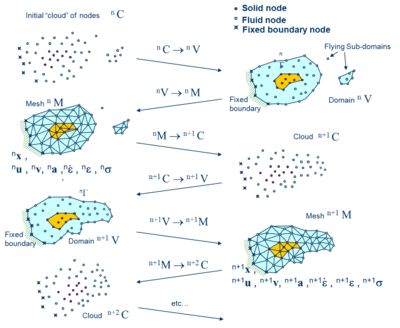Figure 1:Sequence of steps to update a cloud´´ of nodes representing a domain containing a fluid and a solid part from time $n$ ( $t=t_{n}$ ) to time $n+2$ ( $t=t_{n}+2\Delta t$ )

3 FIC/FEM formulation for a Lagrangian continuum

3.1 Governing equations

The equations to be solved are the standard ones in Lagrangian continuum mechanics .

Momentum

 $\rho {\frac {\partial v_{i}}{\partial t}}={\frac {\partial {\sigma }_{ij}}{\partial x_{j}}}+$$b_{i}{\mbox{ }}{\mbox{ }}{\mbox{ }}in{\mbox{ }}{\mbox{ }}V$
(1)

Pressure-velocity relationship

 ${\frac {1}{K}}{\frac {\partial p}{\partial t}}-{\frac {\partial v_{i}}{\partial x_{i}}}=0{\mbox{ }}{\mbox{ }}{\mbox{ }}in{\mbox{ }}{\mbox{ }}V$
(2)

In above equations $v_{i}$ is the velocity along the $i$th global (cartesian) axis, $p$ is the pressure (assumed to be positive in tension) $\rho$ and $K$ are the density and bulk modulus of the material, respectively, $b_{i}$ and ${\sigma }_{ij}$ are the body forces and the (Cauchy) stresses. Eqns (1) and (2) are completed with the constitutive relationships :

Incompressible continuum

 ${}^{t+1}{\sigma }_{ij}=2\mu {\dot {\epsilon }}_{ij}+$$^{t+1}p{\delta }_{ij}$
(3)

Compressible/quasi-incompressible continuum

 ${}^{t+1}{\sigma }_{ij}=^{t}{\overset {\mbox{ˆ}}{\sigma }}_{ij}+$$2\mu {\dot {\epsilon }}_{ij}+\lambda {\dot {\epsilon }}_{ii}{\delta }_{ij}$
(4a)

where ${\overset {\mbox{ˆ}}{\sigma }}_{ij}$ are the component of the Cauchy stress tensor $\left[{\overset {\mbox{ˆ}}{\sigma }}\right]$

 $[{\overset {\mbox{ˆ}}{\sigma }}]={\frac {1}{J}}{\boldsymbol {F}}^{T}{\boldsymbol {SF}}$
(4b)

where ${\textstyle S}$ is the second Piola-Kirchhoff stress tensor, ${\textstyle F}$ is the deformation gradient tensor and ${\textstyle F}$ . Parameters $\mu$ and $\lambda$ take the following values for a fluid or solid material:

Fluid $\mu$ : viscosity; $\lambda =\Delta tK-{\frac {2\mu }{3}}$

Solid $\mu ={\frac {\Delta tG}{J}}$ ; $\lambda ={\frac {2G\nu \Delta t}{J(1-2\nu )}}$ , where $\nu$ is the Poisson ratio, ${\textstyle G}$ is the shear modulus and $\Delta t$ the time increment.

In eqns (3) and (4), ${\dot {\epsilon }}_{ij}$ is the rate of deformation and ${\delta }_{ij}$ is the Kronecker delta. ${}^{t}(\cdot )$ denotes values at time ${\textstyle t}$.

Note that ${}^{t}{\overset {\frown }{\sigma }}_{ij}=0$ for a fluid in eqn.(4a), as the stresses depend on the rates of deformation only.

Indexes in eqns (1)-(4) range from $i,j=1,n_{d}$ , where $n_{d}$ is the number of space dimensions. These equations are completed with the standard boundary conditions of prescribed velocities and surface tractions in the mechanical problem [8-13].

3.2 Discretization of the equations

A key problem in the numerical solution of eqns (1)-(4) is the satisfaction of the mass balance condition for the fully incompressible case (i.e. $K=\infty$ in eqn (2)). A number of procedures to solve this problem exists in the finite element literature [25,27]. In our approach we use a stabilized formulation based in the so-called finite calculus (FIC) procedure [9,14,20-23]. The essence of this method is the solution of a modified mass balance equation which is written as

 ${\frac {1}{K}}{\frac {\partial p}{\partial t}}-{\frac {\partial v_{i}}{\partial x_{i}}}+$$\sum _{i=1}^{3}\tau {\frac {\partial q}{\partial x_{i}}}\left[{\frac {\partial p}{\partial x_{i}}}+{\pi }_{i}\right]=0$
(5)

where ${\textstyle q}$ are weighting functions, $\tau$ is a stabilization parameter given by [9,14,21-23]

 $\tau ={\left({\frac {2\rho \vert v\vert }{h}}+{\frac {8\mu }{3h^{2}}}\right)}^{-1}$
(6)

In the above, ${\textstyle h}$ is a characteristic length of each finite element and $\vert v\vert$ is the modulus of the velocity vector. In eqn (5) ${\pi }_{i}$ are auxiliary pressure projection variables chosen so as to ensure that the second term in eqn (5) can be interpreted as weighted sum of the residuals of the momentum equations and therefore it vanishes for the exact solution. The set of governing equations is completed by adding the following constraint equation .

 ${\int }_{V}\tau w_{i}\left({\frac {\partial p}{\partial x_{i}}}+{\pi }_{i}\right)dV=0{\mbox{ }}i=1,n_{d}{\mbox{ }}{\mbox{ }}({\mbox{no}}{\mbox{ }}{\mbox{sum}}{\mbox{ }}{\mbox{in}}{\mbox{ }}{\mbox{ }}i)$
(7)

where $w_{i}$ are arbitrary weighting functions.

The rest of the integral equations are obtained by applying the weighted residual technique to the governing eqns (1), (2), (3) and (5) and the boundary conditions [9,12,14,18,22,26].

We interpolate next in the finite element fashion the set of problem variables. For 3D problems these are the three velocities $v_{i}$ , the pressure $p$ and the three pressure gradient projections ${\pi }_{i}.$ In our work we use equal order linear interpolation for all variables over meshes of 3-noded triangles (in 2D) and 4-noded tetrahedra (in 3D). The resulting set of discretized equations using the standard Galerkin technique has the following form

Momentum

 ${\boldsymbol {M{\dot {\overline {v}}}}}+{\boldsymbol {K{\overline {v}}}}+$${\boldsymbol {G{\overline {p}}}}={\boldsymbol {f}}$
(8)

Pressure-velocity relationship

 ${\boldsymbol {{\overline {M}}{\dot {\overline {p}}}}}+{\boldsymbol {L{\overline {p}}}}-$${\boldsymbol {G{\overline {v}}}}+{\boldsymbol {Q}}{\mbox{ }}{\boldsymbol {\overline {\pi }}}{\mbox{ }}=0$
(9)

 ${\boldsymbol {{\overset {\frown }{M}}{\overline {\pi }}}}{\mbox{ }}+$${\boldsymbol {Q}}^{T}{\boldsymbol {\overline {p}}}={\boldsymbol {0}}$
(10)

In eqns (8)-(10) ${\overline {\left(\cdot \right)}}$ denotes nodal variables, ${\textstyle {\overset {\cdot }{\overline {\left(\cdot \right)}}}=}$${\frac {\partial }{\partial t}}{\overline {(\cdot )}}$. The matrices and vectors are given in [14,18].

The solution in time of eqns (8)-(10) can be performed using any time integration scheme typical of the updated Lagrangian FEM . A basic algorithm following the conceptual process described in Section 2 is presented in Box I.

Box I. Basic PFEM algorithm for a Lagrangian continuum

 1. LOOP OVER TIME STEPS, ${\textstyle t=1}$, NTIME Known values ${\textstyle ^{t}{\bar {\boldsymbol {x}}},{}^{t}{\bar {\boldsymbol {v}}},{}^{t}{\bar {\boldsymbol {p}}},{}^{t}{\bar {\boldsymbol {\pi }}},{}^{t}{\bar {T}},{}^{t}\mu ,{}^{t}{\boldsymbol {f}},{}^{t}\mathbf {q} ,{}^{t}C,{}^{t}V,{}^{t}M}$ 2. LOOP OVER NUMBER OF ITERATIONS, ${\textstyle i=1}$, NITER ${\textstyle \bullet }$ Compute nodal velocities by solving Eq.(8) ${\textstyle \displaystyle \left[{\frac {1}{\Delta t}}\mathbf {M} +\mathbf {K} \right]{}^{t+1}{\bar {\boldsymbol {v}}}^{i+1}={}^{t+1}\mathbf {f} -{\boldsymbol {G}}^{t+1}{\boldsymbol {p}}^{i}+{\frac {1}{\Delta t}}\mathbf {M} {}^{t}{\bar {\boldsymbol {v}}}}$ ${\textstyle \bullet }$ Compute nodal pressures from Eq.(9) ${\textstyle \displaystyle \left[{\frac {1}{\Delta t}}{\bar {\boldsymbol {M}}}-{\boldsymbol {L}}\right]{}^{t+1}{\bar {\boldsymbol {p}}}^{i+1}=\mathbf {G} {}^{T}{}^{t+1}{\bar {\boldsymbol {v}}}^{i+1}+\mathbf {Q} {}^{t+1}{\bar {\boldsymbol {\pi }}}^{i}+{\frac {1}{\Delta t}}{\bar {\boldsymbol {M}}}{}^{t}{\bar {\boldsymbol {p}}}}$ ${\textstyle \bullet }$ Compute nodal pressure gradient projections from Eq.(10) ${\textstyle {}^{n+1}{\bar {\boldsymbol {\pi }}}^{i+1}=-{\hat {\boldsymbol {M}}}_{D}^{-1}\left[\mathbf {Q} ^{T}\right]{}^{t+1}{\bar {\boldsymbol {p}}}^{i+1}{\begin{array}{ccc}{}&{,}&{{\hat {\boldsymbol {M}}}_{D}=diag\left[{\hat {\boldsymbol {M}}}_{D}\right]}\end{array}}}$ ${\textstyle \bullet }$ Update position of analysis domain nodes: ${\textstyle {}^{t+\Delta t}{\bar {\boldsymbol {x}}}^{i+1}={}^{t}\mathbf {x} ^{i}+{}^{t+\Delta t}\mathbf {v} ^{i+1}\Delta t}$ Define new “cloud” of nodes ${\textstyle {}^{t+1}C^{i+1}}$ ${\textstyle \bullet }$ Update strain rate and strain values ${\textstyle \bullet }$ Update stress values Check convergence ${\textstyle \rightarrow }$ NO ${\textstyle \rightarrow }$ Next iteration ${\textstyle i\to i+1}$ ${\textstyle \downarrow }$ YES Next time step ${\textstyle t\to t+1}$ ${\textstyle \bullet }$ Identify new analysis domain boundary: ${\textstyle {}^{t+1}V}$ ${\textstyle \bullet }$ Generate mesh:${\textstyle {}^{t+1}M}$ Go to 1

4 Generation of a new mesh

A key point for the success of the PFEM is the fast regeneration of a mesh at every time step on the basis of the position of the nodes in the space domain. In our work the mesh is generated using the so called extended Delaunay tesselation (EDT) presented in .

The CPU time required for meshing grows linearly with the number of nodes. The CPU time for solving the equations exceeds that required for meshing as the number of nodes increases. As a general rule for large 3D problems meshing consumes around ${\textstyle 15\%}$ of the total CPU time per time step, while the solution of the equations (with typically 3 iterations per time step) and the system assembly consume approximately ${\textstyle 70\%}$ and ${\textstyle 15\%}$ of the CPU time per time step, respectively. These figures refer to analyses in a single processor Pentium IV PC and prove that the generation of the mesh has an acceptable cost in the PFEM. Indeed considerable speed can be gained using parallel computing techniques.

5 Identification of boundary surfaces

One of the main tasks in the PFEM is the correct definition of the boundary domain. Boundary nodes are sometimes explicitly identified. In other cases, the total set of nodes is the only information available and the algorithm must recognize the boundary nodes (Figure 2).

In our work we use an extended Delaunay partition for recognizing boundary nodes . Considering that the nodes follow a variable $h(x)$ distribution, where $h(x)$ is typically the minimum distance between two nodes. All nodes on an empty sphere with a radius greater than $\alpha h$, are considered as boundary nodes. In practice $\alpha$ is a parameter close to, but greater than one. Values of $\alpha$ ranging around 1.3 have been found to be optimal in all examples analyzed. This criterion is coincident with the Alpha Shape concept .

Once a decision has been made concerning which nodes are on the boundaries, the boundary surface is defined by all the polyhedral surfaces (or polygons in 2D) having all their nodes on the boundary and belonging to just one polyhedron.

The method also allows one to identify isolated fluid particles outside the main fluid domain. These particles are treated as part of the external boundary where the pressure is fixed to the atmospheric value. We recall that each particle is a material point characterized by the density of the solid or fluid domain to which it belongs. The mass lost when a boundary element is eliminated due to departure of a node from the analysis domain is regained when the node falls down and a new boundary element is created by the Alpha Shape algorithm.

The boundary recognition method is useful for detecting contact conditions between the fluid domain and a boundary, as well as between different solids as detailed in the next section.

We emphasize that the key differences between the PFEM and the classical FEM are the remeshing technique and the identification of the domain boundary at each time step.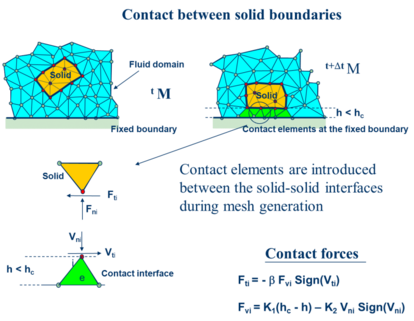Figure 2:Modelling of contact conditions at a solid-solid interface with the PFEM.

6 Treatment of contact conditions in the PFEM

6.1 Contact between the fluid and a fixed boundary

Known velocities at boundaries in the PFEM are prescribed in strong form to the boundary nodes. These nodes might belong to fixed external boundaries or to moving boundaries linked to the interacting solids. Contact between fluid particles and fixed boundaries is accounted for by the incompressibility condition which naturally prevents fluid nodes to penetrate into the solid boundaries [6,9,14].

6.2 Contact between solid-solid interfaces

The contact between two solid interfaces is treated by introducing a layer of contact elements between the two interacting solid interfaces. This layer is automatically created during the mesh generation step by prescribing a minimum distance $\left(h_{c}\right)$ between two solid boundaries. If the distance exceeds the minimum value $\left(h_{c}\right)$ then the generated elements are treated as fluid elements. Otherwise the elements are treated as contact elements where a relationship between the tangential and normal forces and the corresponding displacement is introduced (Figure 2) [6,10].

This algorithm allows us to identify and model complex frictional contact conditions between two or more interacting bodies moving in water in an extremely simple manner. The algorithm can also be used effectively to model frictional contact conditions between rigid or elastic solids in structural mechanics applications .

7 Modeling of bed erosion

Prediction of bed erosion and sediment transport in open channel flows are important tasks in river and environmental engineering. Bed erosion can lead to instabilities of the river basin slopes. It can also undermine the foundation of bridge piles thereby favouring structural failure. Modeling of bed erosion is also relevant for predicting the evolution of surface material dragged in earth dams in overspill situations. Bed erosion is one of the main causes of environmental damage in floods.

In recent works we have proposed an extension of the PFEM to model bed erosion [10,14]. The erosion model is based on the frictional work at the bed surface originated by the shear stresses in the fluid. The resulting erosion model resembles Archard law typically used for modeling abrasive wear in surfaces under frictional contact conditions .

The algorithm for modeling bed erosion is the following:

1. Compute at the bed surface the resultant tangential stress induced by the fluid motion.
2. Compute the frictional work ${\textstyle {}^{n}W_{f}}$ originated by the tangential stresses at the bed surface.
3. The onset of erosion at a bed point occurs when ${\textstyle {}^{n}W_{f}}$ exceeds a critical threshold value ${\textstyle W_{c}}$ .
4. If ${\textstyle {}^{n}W_{f}>W_{c}}$ at a bed node, then the node is detached from the bed region and it is allowed to move with the fluid. Also, the mass of the patch of bed elements surrounding the bed node is transferred to the new fluid node. This mass is subsequently transported with the fluid.

Figure 3 shows an schematic view of the bed erosion algorithm described.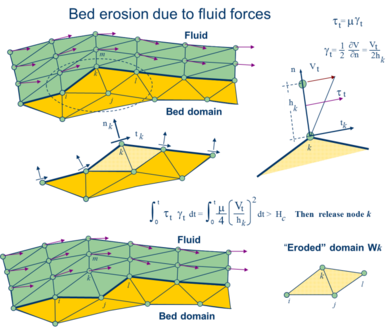Figure 3:Modeling of bed erosion with the PFEM by dragging of bed material.

Sediment deposition can be modeled by an inverse process. Hence, a suspended node adjacent to the bed surface with a velocity below a threshold value is attached to the bed surface.

Examples of the bed erosion algorithm for modeling excavation and rock cutting problems are presented in .

8 Examples

8.1 Dragging of rocks by a water stream

Predicting the critical speed at which a rock will be dragged by a water stream is of great importance in many problems in hydraulic, harbour, civil and environmental engineering.

The PFEM has been successfully applied to the study of the motion of a 1Tn quasi-spherical rock due to a water stream. The rock lays on a collection of rocks that are kept rigid.

Frictional conditions between the analyzed rock and the rest of the rocks have been assumed.

Figure 4a shows that a water stream of 1m/s is not able to displace the individual rock. An increase of the water speed to 2m/s induces the motion of the rock as shown in Figure 4b.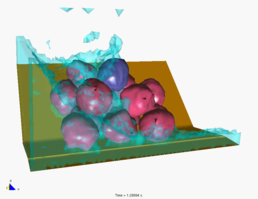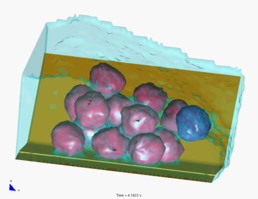(a) Water speed of 1m/s. The individual rock can not be dragged by the stream (b) Water speed of 2m/s. The individual rock is dragged by the stream Figure 4: Drag of a 1 Tn rock under a water stream at speeds of a) 1m/s and b) 2m/s.

8.2 Impact of sea waves on piers and breakwaters

Figure 5 shows the analysis of the effect of breaking waves on two different sites of a breakwater containing reinforced concrete blocks (each one of ${\textstyle 4x4x4}$ mts). The figures correspond to the study of Langosteira harbour in A Coruña, Spain using PFEM.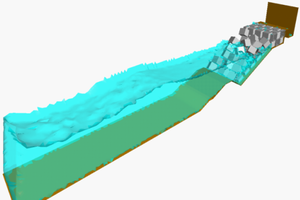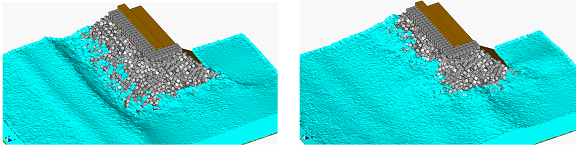Figure 5: Breaking waves on breakwater slopes containing reinforced concrete blocks.

8.3 Soil erosion problems

Figure 6a shows the capacity of the PFEM for modelling soil erosion, sediment transport and material deposition in a river bed. The soil particles are first detached from the bed surface under the action of the jet stream. Then they are transported by the flow and eventually fall down due to gravity forces into the bed surface at a downstream point.

Figure 6b shows the progressive erosion of the unprotected part of a breakwater slope in the Langosteira harbour in A Coruña, Spain. The non protected upper shoulder zone is progressively eroded as it is hit by the sea waves.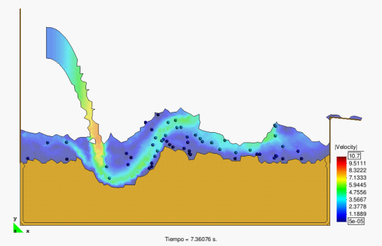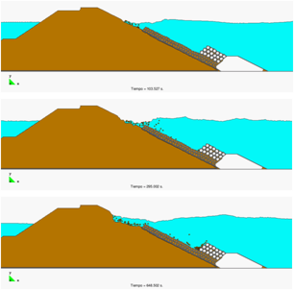(a) (b) Figure 6: (a) Erosion, transport and deposition of soil particles at a river bed due to an impacting jet stream (b) Erosion of an unprotected shoulder of a breakwater due to sea waves

8.4 Falling of a lorry into the sea by sea wave erosion of the road slope

Figure 7 shows a representative example of the progressive erosion of a soil mass adjacent to the shore due to sea waves and the subsequent falling into the sea of a 2D object representing the section of a lorry. The object has been modeled as a rigid solid.

This example and the previous ones, although still quite simple and schematic, show the possibilities of the PFEM for modeling complex FSSI problems involving soil erosion, free surface waves and rigid/deformable structures.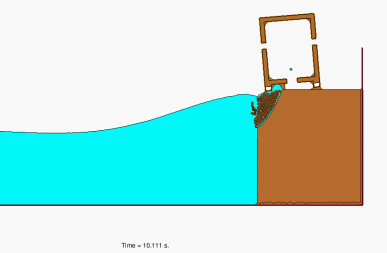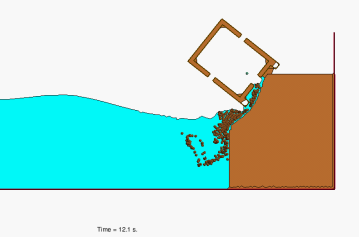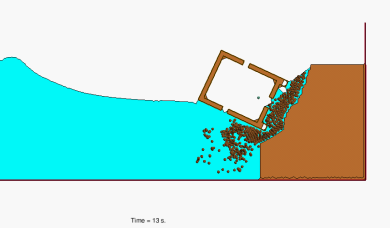Figure 7: Erosion of a soil mass due to sea waves and the subsequent falling into the sea operating in a road adjacent to the sea lorry

8.5 Impact of waves over transport vehicles in harbour

Figures 8 and 9 show two examples of the study of the impact of large waves on lorries operating in roads adjacent to a breakwater.

Figure 8 shows the effect of a wave of 20mts amplitude on a 20 Tns lorry adjacent to a breakwater shoulder.

Figure 9 displays the impact of an overtopping wave on the same lorry placed on a road behing a breakwater.

8.6 Simulation of sinking of ships

The PFEM can be effectively applied for simulating the sinking of ships under a variety of scenarios.

Figure 10 shows images of the 2D simulation of the sinking of a cargo vessel induced by a breach in the bow region.

Figure 11 displays a 3D simulation of the skinking of a simple fisherman boat induced by a hole in the side of the hull.

These examples evidence the potential of PFEM for the study of the sinking of ships.

8.7 Colision of boat with ice blocks

Figures 12 and 13 show two examples of the application of PFEM to the study of the collision of a ship with ice blocks.

Figure 12 shows snapshots of the motion of a boat which collides with several ice blocks.

Figure 13 displays the interaction between a boat and two ice slabs that trap the boat in their motion.

We note that the boat and the ice blocks have been modelled as rigid bodies in these examples. Indeed, the deformation of the ship strucutre due to the ice-ship interaction forces cand be accounted for in the analysis.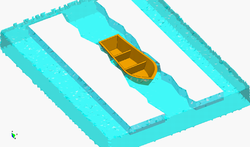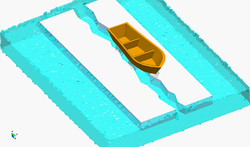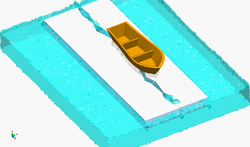Figure 13: Simulation of the interaction of two adjacent ice slabs and boat. The ice slabs move towards the boat that ends up out of the water and over the slabs.

9 Conclusions

The particle finite element method (PFEM) is a promising numerical technique for solving fluid-soil-structure interaction (FSSI) problems in naval, marine and harbour engineering involving large motion of fluid and solid particles, surface waves, water splashing, frictional contact situations between fluid-solid and solid-solid interfaces and bed erosion, among other complex phenomena. The success of the PFEM lies in the accurate and efficient solution of the equations of an incompressible continuum using an updated Lagrangian formulation and a stabilized finite element method allowing the use of low order elements with equal order interpolation for all the variables. Other essential solution ingredients are the efficient regeneration of the finite element mesh, the identification of the boundary nodes using the Alpha-Shape technique and the simple algorithm to treat frictional contact conditions and erosion/wear at fluid-solid and solid-solid interfaces via mesh generation. The examples presented have shown the potential of the PFEM for solving a wide class of practical FSSI problems in naval, marine and harbour engineering.

10 Acknowledgements

This research was partially supported by projects SAFECON and REALTIME of the European Research Council of the European Commission (EC).

11 References

 Idelsohn, S.R., Oñate, E., Del Pin, F. & Calvo, N., Lagrangian formulation: the only way to solve some free-surface fluid mechanics problems, 5th World Congress on Comput. Mechanics, H.A. Mang, F.G. Rammerstorfer & J. Eberhardsteiner (Eds), July 7-12, Viena, Austria, 2002.

 Idelsohn, S.R., Oñate, E. & Del Pin, F., A lagrangian meshless finite element method applied to fluid-structure interaction problems, Comput. and Struct., 81, pp. 655-671, 2003.

 Idelsohn, S.R., Oñate, E., Calvo, N. & Del Pin, F., The meshless finite element method, Int. J. Num. Meth. Engng., 58(6), pp. 893-912, 2003.

 Idelsohn, S.R., Calvo, N. & Oñate, E., Polyhedrization of an arbitrary point set, Comput. Method Appl. Mech. Engng., 192(22-24), pp. 2649-2668, 2003.

 Idelsohn, S.R., Oñate, E. & Del Pin, F., The particle finite element method: a powerful tool to solve incompressible flows with free-surfaces and breaking waves, Int. J. Num. Meth. Engng,. 61, pp. 964-989, 2004.

 Oñate, E., Idelsohn, S.R., Del Pin, F. & Aubry, R., The particle finite element method. An overview, Int. J. Comput. Methods, 1(2), pp. 267-307, 2004b.

 Aubry, R., Idelsohn, S.R. & Oñate, E., Particle finite element method in fluid mechanics including thermal convection-diffusion, Computer & Structures, 83(17-18), pp. 1459-1475, 2005.

 Idelsohn, S.R., Oñate, E., Del Pin, F. & Calvo, N., Fluid-structure interaction using the particle finite element method, Comput. Meth. Appl. Mech. Engng., 195, pp. 2100-2113, 2006.

 Oñate, E., García, J., Idelsohn, S.R. & Del Pin, F., FIC formulations for finite element analysis of incompressible flows. Eulerian, ALE and Lagrangian approaches, Comput. Meth. Appl. Mech. Engng., 195(23-24), pp. 3001-3037, 2006.

 Oñate, E., Celigueta, M.A. & Idelsohn, S.R., Modeling bed erosion in free surface flows by the Particle Finite Element Method, Acta Geotechnia, 1(4), 237-252, 2006.

 Del Pin, F., Idelsohn, S.R., Oñate, E. & Aubry, R., The ALE/Lagrangian particle finite element method: A new approach to computation of free-surface flows and fluid-object interactions, Computers & Fluids, 36, pp. 27-38, 2007.

 Idelsohn, S.R., Marti, J., Limache, A. & Oñate, E. Unified Lagrangian formulation for elastic solids and incompressible fluids: Application to fluid-structure interaction problems via the PFEM, Comput Methods Appl Mech Engrg., 197, pp. 1762-1776, 2008.

 Larese, A., Rossi, R., Oñate, E. & Idelsohn, S.R., Validation of the Particle Finite Element Method (PFEM) for free surface flows, Engng. Computations, 25(4), pp. 385-425, 2008.

 Oñate, E., Idelsohn, S.R., Celigueta, M.A. & Rossi, R., Advances in the particle finite element method for the analysis of fluid-multibody interaction and bed erosion in free surface flows, Comput. Meth. Appl. Mech. Engng., 197(19-20), 1777-1800, 2008.

 Idelsohn, S.R., Mier-Torrecilla, M. & Oñate, E., Multi-fluid flows with the Particle Finite Element Method, Comput Methods Appl Mech Engrg., 198, pp. 2750-2767, 2009.

 Carbonell, J.M,. Oñate, E. & Suárez, B., Modeling of ground excavation with the Particle Finite Element Method, J. of Engineering Mechanics (ASCE), 136(4), pp. 455- 463, 2010.

 Oñate, E., Rossi, R., Idelsohn, S.R. & Butler, K., Melting and spread of polymers in fire with the particle finite element method, Int. J. Numerical Methods in Engng., 81(8), 1046-1072, 2010.

 Oñate, E., Celigueta, M.A., Idelsohn, S.R., Salazar, F. & Suárez B., Possibilities of the particle finite element method for fluid–soil–structure interaction problems, Comput. Mech., 48, pp. 307-318, 2011.

 Edelsbrunner, H. & Mucke, E.P., Three dimensional alpha shapes, ACM Trans. Graphics 13, pp. 43-72, 1999.

 Oñate, E., Derivation of stabilized equations for advective-diffusive transport and fluid flow problems, Comput. Meth. Appl. Mech. Engng., 151, pp. 233-267, 1998.

 Oñate, E., Possibilities of finite calculus in computational mechanics, Int. J. Num. Meth. Engng. 60(1), pp. 255-281, 2004.

 Oñate, E. & García, J., A finite element method for fluid-structure interaction with surface waves using a finite calculus formulation, Comp. Meth. Appl. Mech. Eng., 191, pp. 635-660, 2001.

 Oñate, E., Valls, A. & García, J., FIC/FEM formulation with matrix stabilizing terms for incompressible flows at low and high Reynold's numbers, Comput. Mech., 38 (4-5), pp. 440-455, 2006.

 Zienkiewicz, O.C. & Taylor, R.L., The finite element method for solid and structural mechanics, Elsevier, 2005.

 Donea, J. & Huerta, A., Finite element method for flow problems, J. Wiley, 2003.

 Zienkiewicz, O.C., Taylor, R.L. & Zhu, J.Z., The finite element method. Its basis and fundamentals, Elsevier, 2005.

 Zienkiewicz, O.C., Taylor, R.L. & Nithiarasu, P., The finite element method for fluid dynamics, Elsevier, 2006.

 Archard, J.F., Contact and rubbing of flat surfaces, J. Appl. Phys. 24(8), pp.981-988, 1953.

Document informationPublished on 23/05/19

DOI: 10.1007/978-94-007-6143-8_4
Licence: CC BY-NC-SA license

Document Score0

Views 24
Recommendations 0